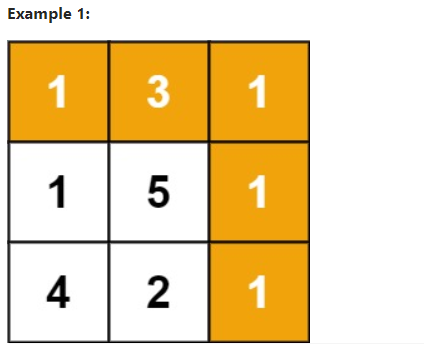# 动态规划 二维 64. Minimum Path Sum

2021/7/20 22:45:42 浏览：

Given a m x n grid filled with non-negative numbers, find a path from top left to bottom right, which minimizes the sum of all numbers along its path.
Note: You can only move either down or right at any point in time.
Example 1:Input: grid = [[1,3,1],[1,5,1],[4,2,1]]
Output: 7
Explanation: Because the path 1 → 3 → 1 → 1 → 1 minimizes the sum.
Example 2:
Input: grid = [[1,2,3],[4,5,6]]
Output: 12
Constraints:
m == grid.length
n == grid[i].length
1 <= m, n <= 200
0 <= grid[i][j] <= 100

``````class Solution {
public:
int minPathSum(vector<vector<int>>& grid) {
int min = INT_MAX;
dfs(grid,0,0,min,0);
return min;
}
void dfs(vector<vector<int>>& grid, int i, int j, int &min, int sum){
sum += grid[i][j];
if(i == grid.size()-1 && j == grid.size()-1){
min = sum < min ? sum : min;
return;
}
if(i + 1 < grid.size())
dfs(grid,i+1,j,min,sum);
if(j +1 < grid.size())
dfs(grid,i,j+1,min,sum);
}
};
``````

``````class Solution {
public:
int minPathSum(vector<vector<int>>& grid) {
vector<vector<int>> dp(grid.size(),vector<int>(grid.size(),0));
for(int i = 0; i < grid.size(); i++){
for(int j = 0; j < grid.size(); j++){
if(i == 0 && j == 0){
dp[i][j] = grid[i][j];
continue;
}
else if(i - 1 >= 0 && j - 1 >= 0){
dp[i][j] = dp[i-1][j] < dp[i][j-1] ? dp[i-1][j] : dp[i][j-1];
}
else if(i == 0)
dp[i][j] = dp[i][j-1];
else if(j == 0)
dp[i][j] = dp[i-1][j];

dp[i][j] += grid[i][j];
}
}
return dp[grid.size()-1][grid.size()-1];
}
};
``````